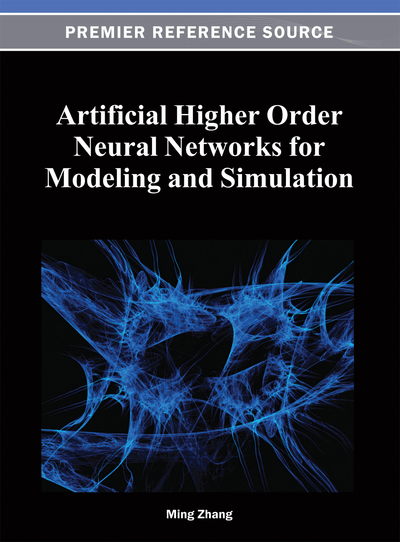# Artificial Higher Order Neural Networks for Modeling Combinatorial Optimization Problems

Yuxin Ding (Harbin Institute of Technology, China)
DOI: 10.4018/978-1-4666-2175-6.ch003
Available
\$37.50
No Current Special Offers

## Abstract

Traditional Hopfield networking has been widely used to solve combinatorial optimization problems. However, high order Hopfiled networks, as an expansion of traditional Hopfield networks, are seldom used to solve combinatorial optimization problems. In theory, compared with low order networks, high order networks have better properties, such as stronger approximations and faster convergence rates. In this chapter, the authors focus on how to use high order networks to model combinatorial optimization problems. Firstly, the high order discrete Hopfield Network is introduced, then the authors discuss how to find the high order inputs of a neuron. Finally, the construction method of energy function and the neural computing algorithm are presented. In this chapter, the N queens problem and the crossbar switch problem, which are NP-complete problems, are used as examples to illustrate how to model practical problems using high order neural networks. The authors also discuss the performance of high order networks for modeling the two combinatorial optimization problems.
Chapter Preview
Top

## 2. High-Order Discrete Hopfield Network

In general, there are two types of Hopfield neural network, Continuous Hopfield Neural Network (CHNN) and Discrete Hopfield Neural Network (DHNN). In this chapter, we focus on DHNN. A DHNN with n neurons can be uniquely represented by an n × n real matrixand an n-dimensional column vector. We denote a DHNN as. DHNN working in serial mode can be described as follows:

(1) where. Equation (2) is the standard energy function of the network.

## Complete Chapter List

Search this Book:
Reset